How Cheenta works to ensure student success?
Explore the Back-Story

# Supremum and Infimum: IIT JAM 2018 Problem 11# Understand the problemWhich of the following is true? (a) sup {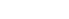}=3 and inf {}=1 (b) lim inf (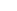)=lim sup ()=(c) sup {}=2 and inf {}=1 (d) lim inf ()= 1 lim sup ()=3

Hint 1:Now the limit points of this set are those points which the set does not attain.So, they might be the sup and inf which are not attained by this set. Basically sup()= max{ limit points,| n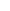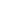} Limit points are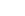and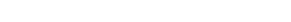Now you can calculate  the supremum?

Hint 2: From the observation of Hint 2 we have  sup= max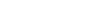Similarly, inf= minlimit points,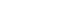Can you calculate that by yourself? Hint 3: inf= min {2,1,2 -}=1 So, option A is correct. Now there is another question regarding  lim sup and lim inf. We can observe that we have mainly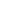subsequences , corresponding to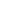is even;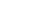=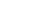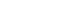Can you calculate the corresponding subsequences  and their limits?

Hint 4: Forwe have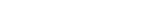ask For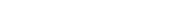askask So, lim sup=max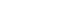Lim inf=minTherefore, Option C is also correct

# Connected Program at Cheenta

The higher mathematics program caters to advanced college and university students. It is useful for I.S.I. M.Math Entrance, GRE Math Subject Test, TIFR Ph.D. Entrance, I.I.T. JAM. The program is problem driven. We work with candidates who have a deep love for mathematics. This program is also useful for adults continuing who wish to rediscover the world of mathematics.

# Understand the problemWhich of the following is true? (a) sup {}=3 and inf {}=1 (b) lim inf ()=lim sup ()=(c) sup {}=2 and inf {}=1 (d) lim inf ()= 1 lim sup ()=3

Hint 1:Now the limit points of this set are those points which the set does not attain.So, they might be the sup and inf which are not attained by this set. Basically sup()= max{ limit points,| n} Limit points areandNow you can calculate  the supremum?

Hint 2: From the observation of Hint 2 we have  sup= maxSimilarly, inf= minlimit points,Can you calculate that by yourself? Hint 3: inf= min {2,1,2 -}=1 So, option A is correct. Now there is another question regarding  lim sup and lim inf. We can observe that we have mainlysubsequences , corresponding tois even;=Can you calculate the corresponding subsequences  and their limits?

Hint 4: Forwe haveask Foraskask So, lim sup=maxLim inf=minTherefore, Option C is also correct

# Connected Program at Cheenta

The higher mathematics program caters to advanced college and university students. It is useful for I.S.I. M.Math Entrance, GRE Math Subject Test, TIFR Ph.D. Entrance, I.I.T. JAM. The program is problem driven. We work with candidates who have a deep love for mathematics. This program is also useful for adults continuing who wish to rediscover the world of mathematics.

# Similar Problems

This site uses Akismet to reduce spam. Learn how your comment data is processed.

### Knowledge Partner